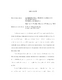## Experimental Characterization of Turbulent Superfluid Helium2010
##### Authors
Paoletti, Matthew S.
Fundamental processes in turbulent superfluid <super>4</super>He are experimentally characterized by refining a visualization technique recently introduced by Bewley <italic>et al</italic>. A mixture of hydrogen and helium gas is injected into the bulk fluid, which produces a distribution of micron-sized hydrogen tracer particles that are visualized and individually tracked allowing for local velocity measurements. Tracer trajectories are complex since some become trapped on the quantized vortices while others flow with the normal fluid. This technique is first applied to study the dynamics of a thermal counterflow. The resulting observations constitute the first direct confirmation of two-fluid motions in He II and provide a quantitative test of the expression for the dependence of the normal fluid velocity, <bold>v</bold><sub>n</sub>, on the applied heat flux, <bold>q</bold>, derived by L. D. Landau in 1941. Nearly 20,000 individual reconnection events are identified for the first time and used to characterize the dynamics by the minimum separation distance, $delta(t)$, between two reconnecting vortices. Dimensional arguments predict that this separation behaves asymptotically as $delta(t) approx A left ( kappa vert t-t_0 vert right ) ^{1/2}$, where $kappa=h/m$ is the quantum of circulation. The major finding of the experiments is strong support for this asymptotic form with $kappa$ as the dominant controlling quantity. Nevertheless there are significant event-to-event fluctuations that are equally well fit by two modified expressions: (a) an arbitrary power-law expression $delta(t)=B vert t-t_0 vert ^{alpha}$ and (b) a correction-factor expression $delta(t)=Aleft (kappa vert t-t_0 vert right ) ^{1/2}(1+c vert t-t_0 vert )$. In light of various physical interpretations we regard the correction-factor expression (b), which attributes the observed deviations from the predicted asymptotic form to fluctuations in the local environment and boundary conditions, as best describing the experimental data. The observed dynamics appear statistically time-reversible, suggesting that an effective equilibrium has been established in quantum turbulence on the time scales investigated. The hydrogen tracers allow for the first measurements of the local velocity statistics of a turbulent quantum fluid. The distributions of velocity in the decaying turbulence are strongly non-Gaussian with 1/v<super>3</super> power-law tails in contrast to the near-Gaussian statistics of homogenous and isotropic turbulence of classical fluids. The dynamics of many vortex reconnection events are examined and simple scaling arguments show that they yield the observed power-law tails.